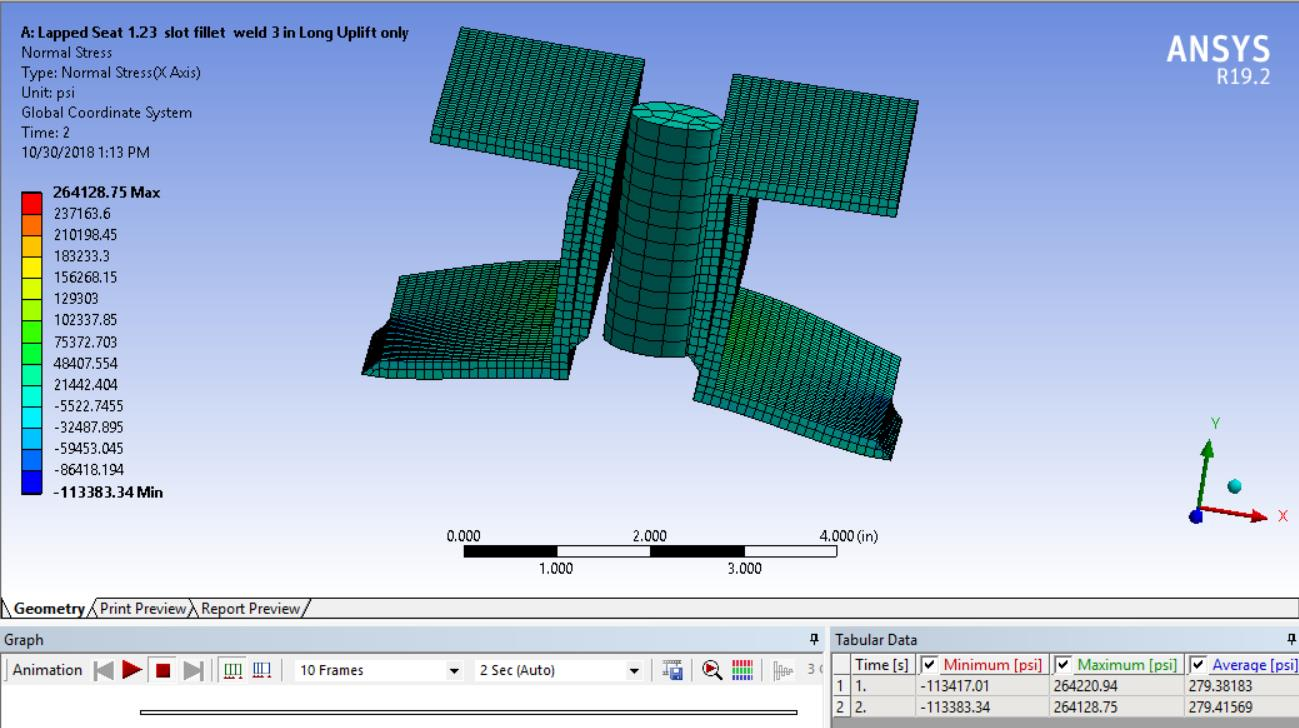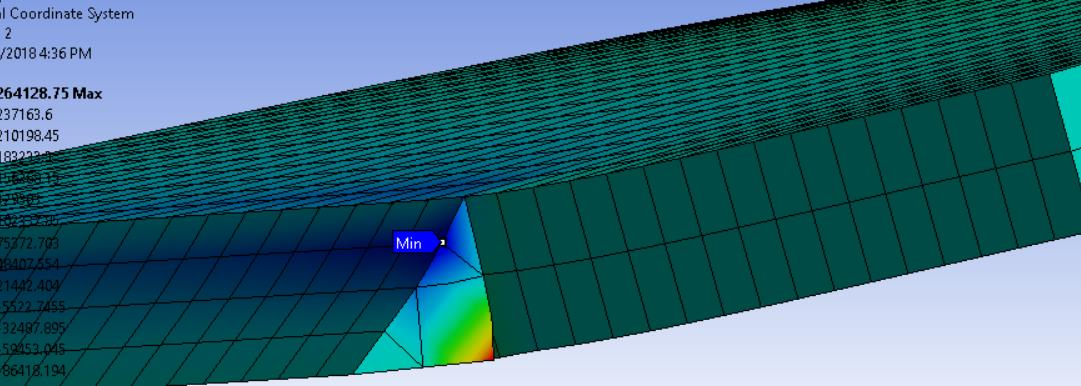## General Mechanical

•sameerfares
Subscriber

Hi All,

I am wondering about the normal stress magnitudes. i applied a 2000 lbf upward force at center of rod. Is the maximum normal stress 264220.94 psi and average stress 279.38 psi? why is the average normal stress much lower than maximum stress? Am I misreading something?

Thanks,

Sam•peteroznewman
Subscriber

Hi Sam,

You care about the Maximum and Minimum Normal Stress in a plot that includes all bodies.  You don't care about the Average. In version 18 and earlier, results didn't even show an average.

An even more useful plot is Equivalent von-Mises Stress because you have a complex 3D state of stress and components of stress in directions other than the X-axis are significant.  You can compare the Equivalent von-Mises stress with the Yield Strength to see if you have exceeded that threshold and the load is predicted to permanently deform the structure.

An example of when an Average Normal Stress might be useful is when you request stress on a surface that represents the cross-section of a part in tension. There may be a variation in stress across the section, but you want to know the average stress so you can multiply by the cross-sectional area to get  a force.

Regards,
Peter

•sameerfares
Subscriber

Hi Peter,

I am question the high value of the stress. 2000 lbf  uplift force to cause 270000 psi. That seems very high stress.

Regards,

Sam

•peteroznewman
Subscriber

Hi Sam,

The 2000 lbf is pulling up in the center, a large distance from the support at the side. The side support is on the thin edge of the part. This creates a large lever due to the distance to the center divided by the thickness of the edge.  That means a large multiplier on the center force to calculate the edge force to support the moment. It seems reasonable to me.

Regards,
Peter

•sameerfares
Subscriber

This is a common connection that we use when the joist or truss is under wind uplift condition. The material  ultimate strength is 65,000 psi and with a yield strength 50,000 psi. Does this mean the material will fail? This has never happened.

Peter: on my yesterday's post regarding the contact surfaces that you fixed for me, i had few questions. one of the i was wondering how you have 5 contact surfaces.

Regards,

Sam

•sameerfares
Subscriber

Peter,

in the picture below , it shows the maximum stress is in the weld. So it is a localized max stress. Is there anything in Ansys that i can use to indicate if the connection will fail?

Regards,

Sam•peteroznewman
Subscriber

Hi Sam,

Open Engineering Data, look for the Plasticity category. Drag and drop Bilinear Isotropic Hardening onto the two materials you are using. One of the inputs in that material model is Yield Strength. That is where you enter 50,000 psi. The other input is Tangent Modulus. If you enter 0 for the Tangent Modulus, that defines what Mechanics of Materials textbooks call an Elastic, Perfectly-Plastic material.

After the solution runs, there will be no stress above the yield stress (except for extrapolation to nodes). You may need smaller elements near the parts of the model that have the highest stress.

There is a material property called Elongation, sometimes called Elongation at Break or Failure.  It's the last value of strain on a Tensile Stress-Strain plot.

To decide if the assembly is predicted to fail, compare Total Strain with Elongation.  If Total Strain > Elongation, then failure is predicted.

You can compute a Factor of Safety as Elongation/Total Strain.  If Factor of Safety < 1, then failure is predicted.

I will look at your 5 face question in the other thread.

Regards,
Peter